# Trees trees binary trees traversals of trees template

• Slides: 19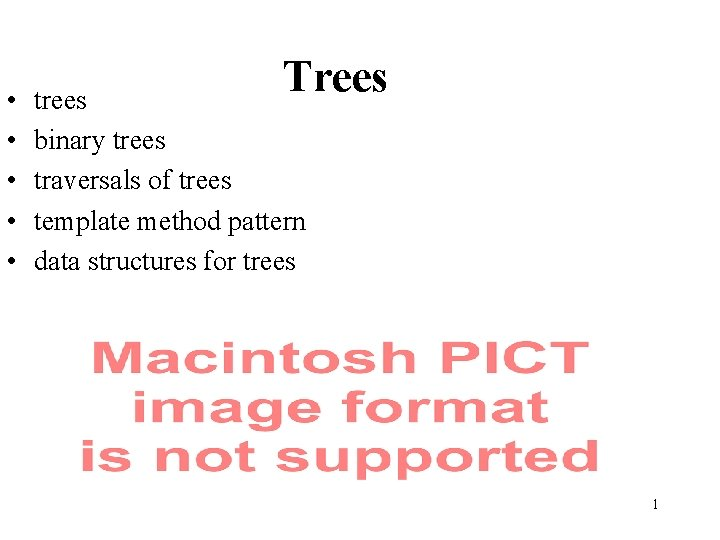• • • Trees trees binary trees traversals of trees template method pattern data structures for trees 1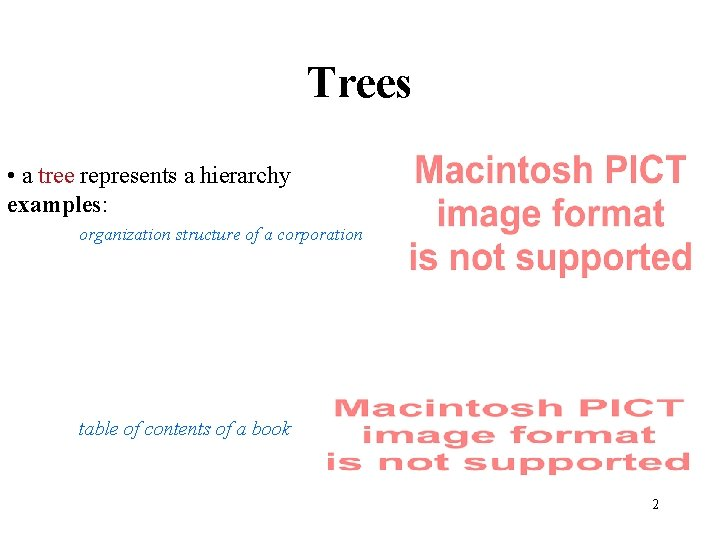Trees • a tree represents a hierarchy examples: organization structure of a corporation table of contents of a book 2Another Example • ` Unix or DOS/Windows file system 34Binary Trees 5Examples of Binary Trees • arithmetic expression • river 6Examples of Binary Trees • decision trees 7Properties of Binary Trees 8ADTs for Trees • generic container methods -size(), is. Empty(), elements() • positional container methods -positions(), swap. Elements(p, q), replace. Element(p, e) • query methods -is. Root(p), is. Internal(p), is. External(p) • accessor methods -root(), parent(p), children(p) • update methods -application specific 9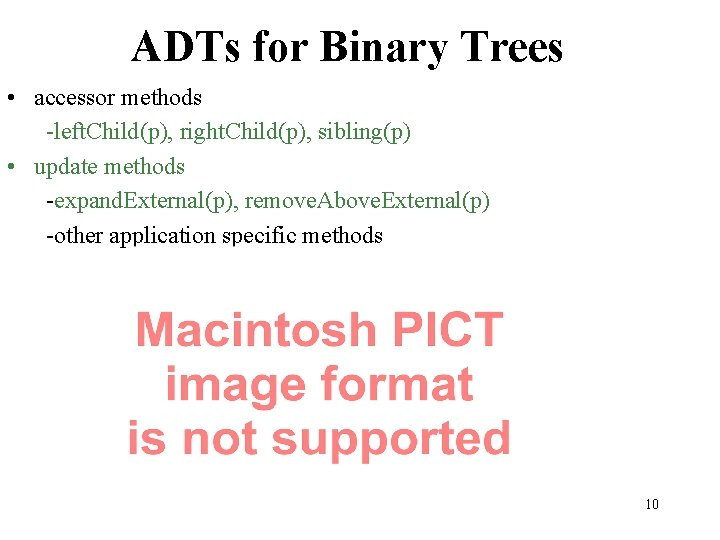ADTs for Binary Trees • accessor methods -left. Child(p), right. Child(p), sibling(p) • update methods -expand. External(p), remove. Above. External(p) -other application specific methods 10Traversing Trees • preorder traversal Algorithm pre. Order(v) “visit” node v for each child w of v do recursively perform pre. Order(w) • reading a document from beginning to end 11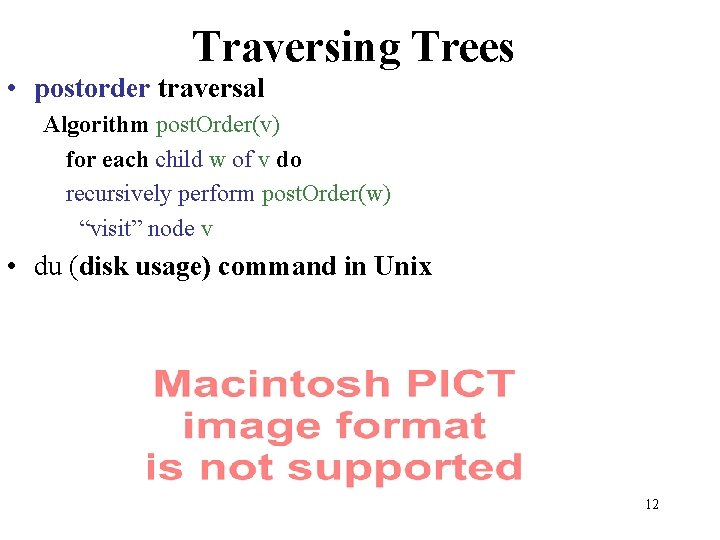Traversing Trees • postorder traversal Algorithm post. Order(v) for each child w of v do recursively perform post. Order(w) “visit” node v • du (disk usage) command in Unix 12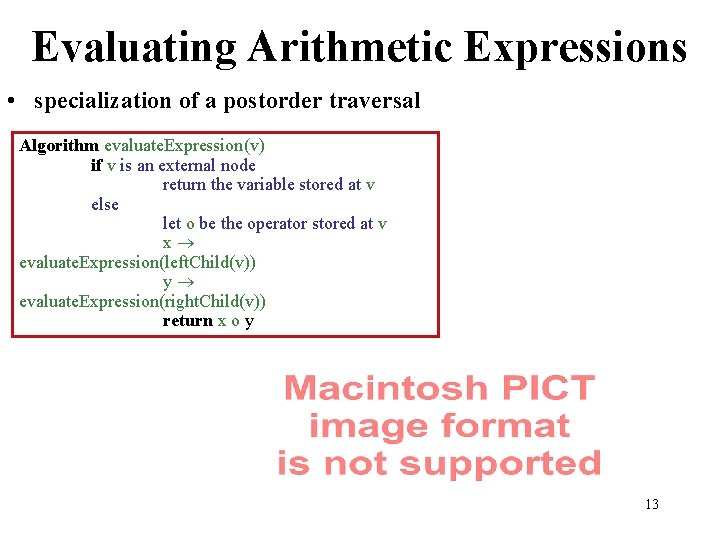Evaluating Arithmetic Expressions • specialization of a postorder traversal Algorithm evaluate. Expression(v) if v is an external node return the variable stored at v else let o be the operator stored at v x evaluate. Expression(left. Child(v)) y evaluate. Expression(right. Child(v)) return x o y 13Traversing Trees • inorder traversal of a binary tree Algorithm in. Order(v) recursively perform in. Order(left. Child(v)) “visit” node v recursively perform in. Order(right. Child(v)) • printing an arithmetic expression specialization of an inorder traversal print “(“ before traversing the left subtree print “)” after traversing the right subtree 14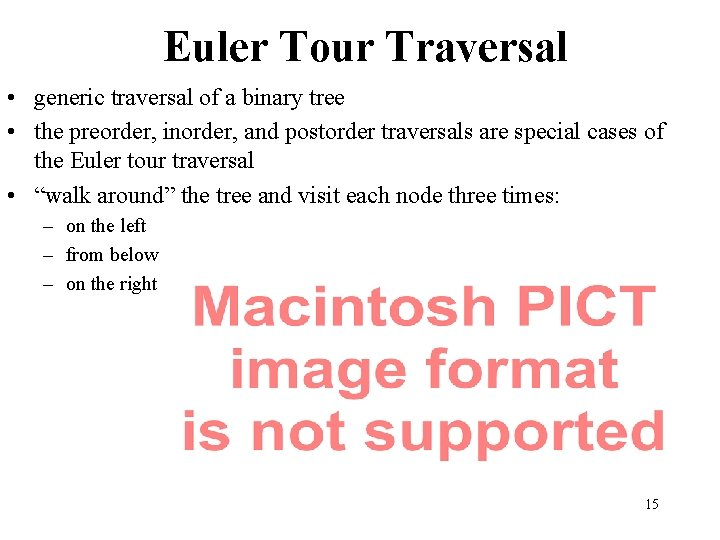Euler Tour Traversal • generic traversal of a binary tree • the preorder, inorder, and postorder traversals are special cases of the Euler tour traversal • “walk around” the tree and visit each node three times: – on the left – from below – on the right 15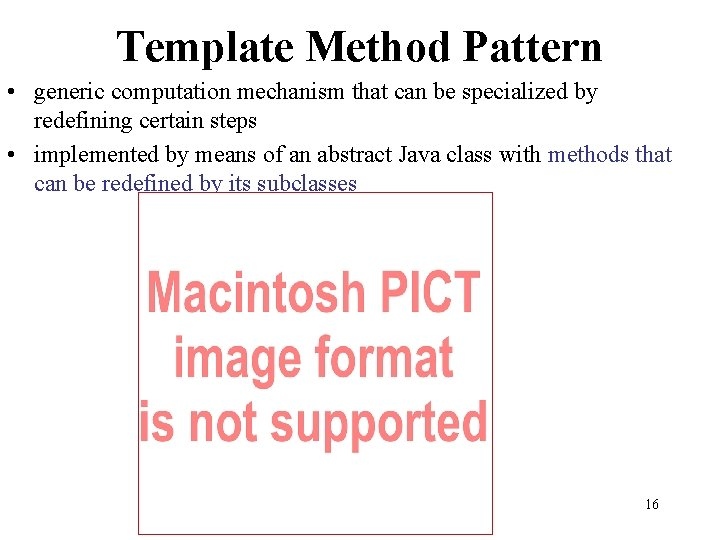Template Method Pattern • generic computation mechanism that can be specialized by redefining certain steps • implemented by means of an abstract Java class with methods that can be redefined by its subclasses 16Specializing the Generic Binary Tree Traversal • printing an arithmetic expression public class Print. Expression. Traversal extends Binary. Tree. Traversal {. . . protected void external(Position p, Traversal. Result r) { System. out. print(p. element()); } protected void left(Position p, Traversal. Result r) { System. out. print("("); } protected void below(Position p, Traversal. Result r) { System. out. print(p. element()); } protected void right(Position p, Traversal. Result r) { System. out. print(")"); } 17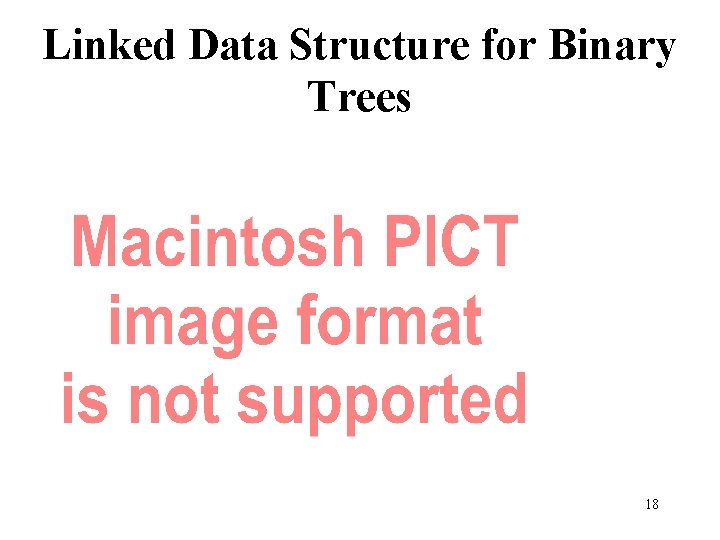Linked Data Structure for Binary Trees 18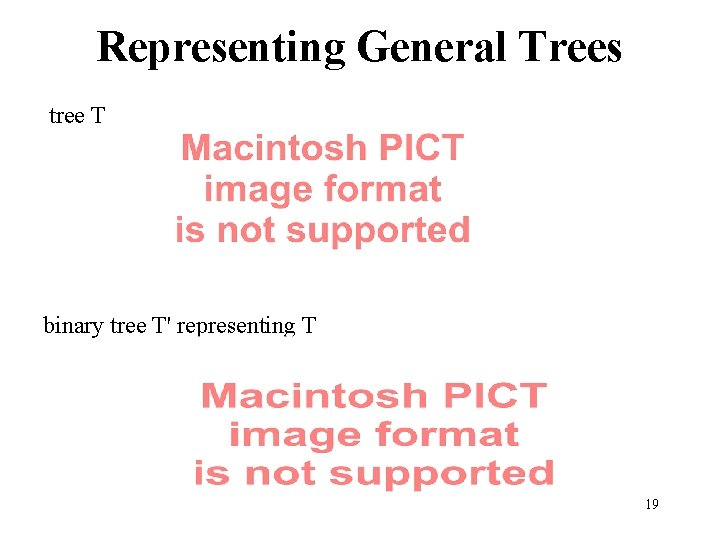Representing General Trees tree T binary tree T' representing T 19